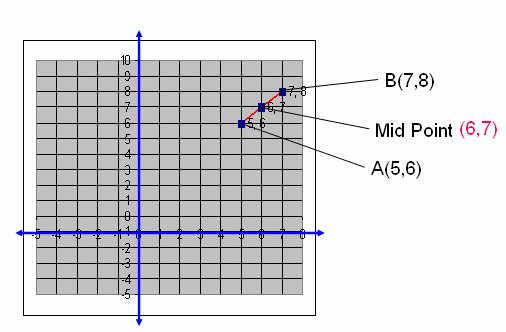Email us to get an instant 20% discount on highly effective K-12 Math & English kwizNET Programs!

#### Online Quiz (WorksheetABCD)

Questions Per Quiz = 2 4 6 8 10

### High School Mathematics - 29.8 Mid Point of the Segment Joining Two Points

 If A(x1 , y1) and B(x2 , y2) are two points, then the mid-point of the segment joining AB is [(x1 + x2)/2 , (y1 + y2)/2] i.e., (Sum of x-coordinates/2 , Sum of y-coordinates/2). Example: Find the mid point of (5,6) and (7,8). Solution: Formula for mid point of the segment joining two points is [(x1 + x2)/2 , (y1 + y2)/2] = [(5 + 7)/2 , (6 + 8)/2] = (12/2 , 14/2) = (6,7) Therefore, the mid point of (5,6) and (7,8) is (6,7). Showing the above problem in the Graph as below.Directions: Find the mid point of the segment joining two points and draw a graph each of the following questions. Also write at least 5 examples of your own.
 Q 1: Find the mid point of the segment joining the points (4,8) and (2,4).(4,2)(3,6)(2,4)(6,3) Q 2: Find the mid point of the segment joining the points (3,4) and (4,5).(4.5,5.5)(4,5)(3.5,4.5)(3,4) Q 3: Find the mid point of the segment joining the points (6,12) and (3,6).(4.5,9)(5,9)(4,9)(5.5,9) Q 4: Find the mid point of the segment joining the points (7,8) and (15,16).(13,14)None of these(12,13)(11,12) Q 5: Find the mid point of the segment joining the points (7,8) and (11,12).(9,8)(10,9)(8,9)(9,10) Q 6: Find the mid point of the segment joining the points (7,5) and (8,6).(7,5)(5,7)None of these(7.5,5.5) Question 7: This question is available to subscribers only! Question 8: This question is available to subscribers only!

#### Subscription to kwizNET Learning System offers the following benefits:

• Unrestricted access to grade appropriate lessons, quizzes, & printable worksheets
• Instant scoring of online quizzes
• Progress tracking and award certificates to keep your student motivated
• Unlimited practice with auto-generated 'WIZ MATH' quizzes